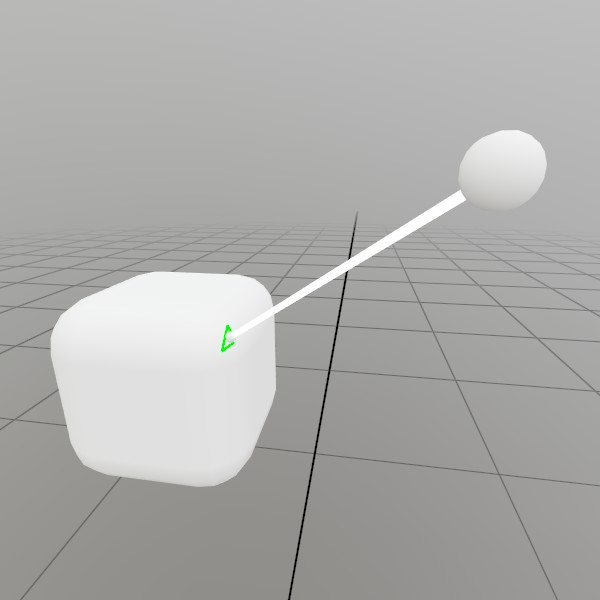# Mesh.Intersect

``````bool Intersect(Ray modelSpaceRay, Ray& modelSpaceAt)
``````

Checks the intersection point of this ray and a Mesh with collision data stored on the CPU. A mesh without collision data will always return false. Ray must be in model space, intersection point will be in model space too. You can use the inverse of the mesh’s world transform matrix to bring the ray into model space, see the example in the docs!

Ray modelSpaceRay Ray must be in model space, the intersection point will be in model space too. You can use the inverse of the mesh’s world transform matrix to bring the ray into model space, see the example in the docs!
Ray& modelSpaceAt The intersection point and surface direction of the ray and the mesh, if an intersection occurs. This is in model space, and must be transformed back into world space later. Direction is not guaranteed to be normalized, especially if your own model->world transform contains scale/skew in it.
RETURNS: bool True if an intersection occurs, false otherwise!
``````bool Intersect(Ray modelSpaceRay, Ray& modelSpaceAt, UInt32& outStartInds)
``````

Checks the intersection point of this ray and a Mesh with collision data stored on the CPU. A mesh without collision data will always return false. Ray must be in model space, intersection point will be in model space too. You can use the inverse of the mesh’s world transform matrix to bring the ray into model space, see the example in the docs!

Ray modelSpaceRay Ray must be in model space, the intersection point will be in model space too. You can use the inverse of the mesh’s world transform matrix to bring the ray into model space, see the example in the docs!
Ray& modelSpaceAt The intersection point and surface direction of the ray and the mesh, if an intersection occurs. This is in model space, and must be transformed back into world space later. Direction is not guaranteed to be normalized, especially if your own model->world transform contains scale/skew in it.
UInt32& outStartInds The index of the first index of the triangle that was hit
RETURNS: bool True if an intersection occurs, false otherwise!

## Examples

### Ray Mesh Intersection

Here’s an example of casting a Ray at a mesh someplace in world space, transforming it into model space, calculating the intersection point, and displaying it back in world space.``````Mesh sphereMesh = Default.MeshSphere;
Mesh boxMesh    = Mesh.GenerateRoundedCube(Vec3.One*0.2f, 0.05f);
Pose boxPose    = new Pose(0,     0,     -0.5f,  Quat.Identity);
Pose castPose   = new Pose(0.25f, 0.21f, -0.36f, Quat.Identity);

public void Update()
{
// Draw our setup, and make the visuals grab/moveable!
UI.Handle("Box",  ref boxPose,  boxMesh.Bounds);
UI.Handle("Cast", ref castPose, sphereMesh.Bounds*0.03f);
boxMesh   .Draw(Default.MaterialUI, boxPose .ToMatrix());
sphereMesh.Draw(Default.MaterialUI, castPose.ToMatrix(0.03f));

// Create a ray that's in the Mesh's model space
Matrix transform = boxPose.ToMatrix();
Ray    ray       = transform
.Inverse
.Transform(Ray.FromTo(castPose.position, boxPose.position));

// Draw a sphere at the intersection point, if the ray intersects
// with the mesh.
if (ray.Intersect(boxMesh, out Ray at, out uint index))
{
sphereMesh.Draw(Default.Material, Matrix.TS(transform.Transform(at.position), 0.01f));
if (boxMesh.GetTriangle(index, out Vertex a, out Vertex b, out Vertex c))
{
Vec3 aPt = transform.Transform(a.pos);
Vec3 bPt = transform.Transform(b.pos);
Vec3 cPt = transform.Transform(c.pos);